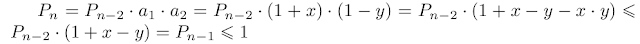# javahotel

Blog do projektu Open Source JavaHotel

## wtorek, 20 października 2009

### Math exercise and proof

(Michał Krych - Analiza Matematyczna część 1)

Assume that we have N positive numbers and the sum of this numbers is not greater than N.Prove that multiplication of this numbers is not greater than 1.Proof
Assume that all number are equal - obvious.
Assume that not all numbers are equal.

Induction proof.
The statement is satisfied for N=1 - obvious.

Inductive step.
Assume that statement holds true for all 1...N-1
Now consider a set of N positive numbers, call their sum as S(n) and assume that S(n) is less or equal N. Call the product if this numbers as P(n).
Because the numbers are not equal at least one should be greater than 1 and at least one should be less than 1.
Call them a1 = 1 + x and a2 = 1 - y.
Consider set of N-2 number (all numbers except a1 and a2) and call its product as P(n-2) and sum as S(n-2)
Than add to this set the number 1 + x - y. This number is positive and call the sum of this numbers (including 1 + x - y) as S(n-1).Call the product as P(n-1). Using the induction hypothesis P(n-1) is less or equal 1.Now let's calculate P(n). Keep in mind that x*y is greater than 0.Q.E.D